# THE BILINEAR TRANSFORM

Z transforms and Fourier transforms are related by the relations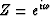and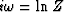.A problem with these relations is that simple ratios of polynomials in Z do not translate to ratios of polynomials in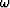and vice versa. The approximation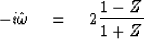(42)
is easily solved for Z as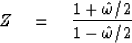(43)
These approximations are often useful. They are truncations of the exact power series expansions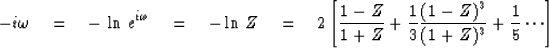(44)
and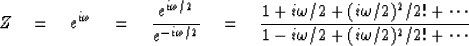(45)

For a Z transform B(Z) to be minimum phase, any root Z0 of 0 = B(Z0) should be outside the unit circle. Since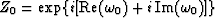and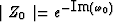, it means that for a minimum phase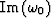should be negative. (In other words,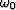is in the lower half-plane.) Thus it may be said thatmaps the exterior of the unit circle to the lower half-plane. By inspection of Figures 20 and 21, it is found that the bilinear approximation (42) or (43) also maps the exterior of the unit circle into the lower half-plane.

 2-20 Figure 20 Some typical points in the Z-plane, the-plane, and the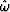-plane.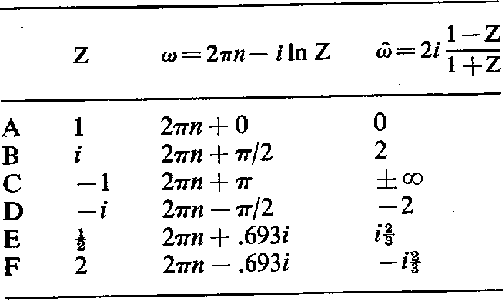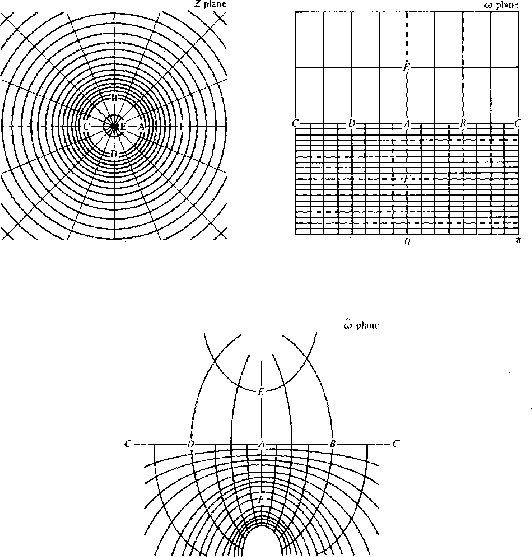2-21
Figure 21
The points of Figure 20 displayed in the Z plane, theplane, and the-plane.

Thus, although the bilinear approximation is an approximation, it turns out to exactly preserve the minimum-phase property. This is very fortunate because if a stable differential equation is converted to a difference equation via (42), the resulting difference equation will be stable. (Many cases may be found where the approximation of a time derivative by multiplication with 1 - Z would convert a stable differential equation into an unstable difference equation.)

A handy way to remember (42) is that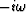corresponds to time differentiation of a Fourier transform and (1 - Z) is the first differencing operator. The (1 + Z) in the denominator gets things centered" at Z1/2

To see that the bilinear approximation is a low-frequency approximation, multiply top and bottom of (42) by Z-1/2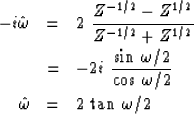(46)
Equation (46) implicitly refers to a sampling rate of one sample per second. Taking an arbitrary sampling rate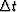, the approximation (46) becomes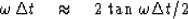(47)
This approximation is plotted in Figure 22. Clearly, the error can be made as small as one wishes merely by sampling often enough; that is, takingsmall enough.

 2-22 Figure 22 The accuracy of the bilinear transformation approximation.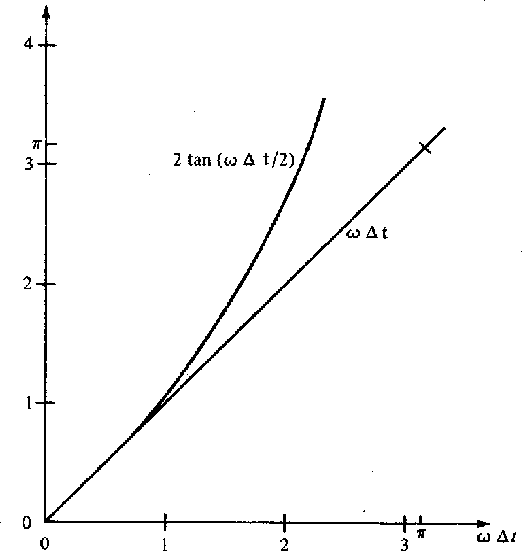From Figure 22 we see that the error will be only a few percent if we choosesmall enough so that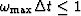. Readers familiar with the folding theorem will recall that it gives the less severe restraint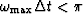. Clearly, the folding theorem is too generous for applications involving the bilinear transform.

Now, by way of example, let us take up the case of a pole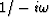at zero frequency. This is integration. For reasons which will presently be clear, we will consider the slightly different pole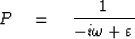(48)
whereis small. Inserting the bilinear transform, we get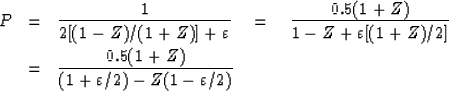(49)
By inspection of (49) we see that the time-domain function is real, and asgoes to zero it takes the form (.5, 1, 1, 1, ...). (Takingpositive forces the step to go out into positive time, whereasnegative would cause the step to rise at negative time.) The properties of this function are summarized in Figure 23.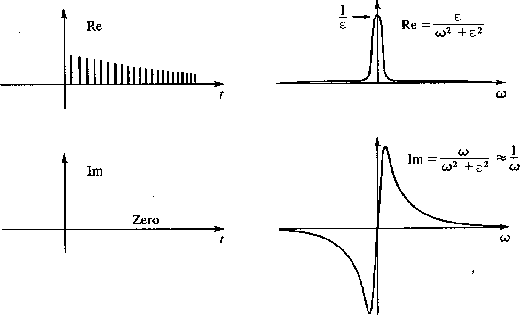2-23
Figure 23
Properties of the integration operator.

## EXERCISES:

1. In the solution to diffusion problems, the factor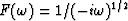often arises as a multiplier. To see the equivalent convolution operation, find a causal, sampled-time representation ft of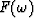by identification of powers of Z in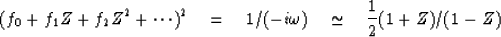Solve numerically for f0 through f7.